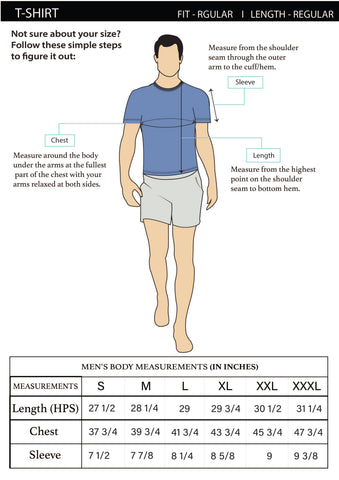English
INR
English
INR

Quick viewQuick view
Rs. 299.00
Rs. 499.00
-40%
Rs. 299.00
Rs. 499.00
-40%
Rs. 299.00
Rs. 499.00
-40%
Rs. 299.00
Rs. 499.00
-40%
Rs. 299.00
Rs. 499.00
-40%
Rs. 299.00
Rs. 499.00
-40%
Rs. 299.00
Rs. 499.00
-40%
Rs. 299.00
Rs. 499.00
-40%
Rs. 299.00
Rs. 499.00
-40%
Rs. 299.00
Rs. 499.00
-40%
Rs. 299.00
Rs. 499.00
-40%
Rs. 299.00
Rs. 499.00
-40%
Rs. 299.00
Rs. 499.00
-40%
Rs. 299.00
Rs. 499.00
-40%
Rs. 299.00
Rs. 499.00
-40%
Rs. 299.00
Rs. 499.00
-40%
Rs. 299.00
Rs. 499.00
-40%
Rs. 299.00
Rs. 499.00
-40%
Rs. 299.00
Rs. 499.00
-40%
Rs. 299.00
Rs. 499.00
-40%
Rs. 299.00
Rs. 499.00
-40%
Rs. 299.00
Rs. 499.00
-40%
Rs. 299.00
Rs. 499.00
-40%
Rs. 299.00
Rs. 499.00
-40%
Rs. 299.00
Rs. 499.00
-40%
Rs. 299.00
Rs. 499.00
-40%
Rs. 299.00
Rs. 499.00
-40%
Rs. 299.00
Rs. 499.00
-40%
Rs. 299.00
Rs. 499.00
-40%
Rs. 299.00
Rs. 499.00
-40%
Rs. 299.00
Rs. 499.00
-40%
Rs. 299.00
Rs. 499.00
-40%
Rs. 299.00
Rs. 499.00
-40%
Rs. 299.00
Rs. 499.00
-40%
Rs. 299.00
Rs. 499.00
-40%
Rs. 299.00
Rs. 499.00
-40%
Rs. 299.00
Rs. 499.00
-40%
Rs. 299.00
Rs. 499.00
-40%
Rs. 299.00
Rs. 499.00
-40%
Rs. 299.00
Rs. 499.00
-40%
Rs. 299.00
Rs. 499.00
-40%
Rs. 299.00
Rs. 499.00
-40%
Rs. 299.00
Rs. 499.00
-40%
Rs. 299.00
Rs. 499.00
-40%
Rs. 299.00
Rs. 499.00
-40%
Rs. 299.00
Rs. 499.00
Rs. 299.00
Rs. 499.00
Rs. 299.00
Rs. 499.00
Rs. 299.00
Rs. 499.00
Rs. 299.00
Rs. 499.00
Rs. 299.00
Rs. 499.00
Rs. 299.00
Rs. 499.00
Rs. 299.00
Rs. 499.00
Rs. 299.00
Rs. 499.00
Rs. 299.00
Rs. 499.00
-40%
Size
S
M
L
XL
XXL
3XL
Color
GREY
RUST
RED
AQUA BLUE
LIGHT GREEN
PRUSSIAN BLUE
PURPLE
TEAL GREEN
VIOLET
Size
Color
View full details
Description

The perfect addition to your summer wardrobe, our lightweight, breathable, and comfortable men's round neck t-shirt is a wardrobe staple for all those hot days. Whether you're lounging around the house or going out for a hike, this t-shirt is the perfect addition to your summer wardrobe. With a light weight, our men's round neck t-shirt is easy to pack and take with you on your next vacation.